Bilbao Crystallographic Server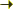Transformation matrix

## The transformation matrix

The transformation matrix P = (P, p) relating two coordinate systems with basis vectors (a, b, c) and (a', b', c') has two parts:
• The linear part: P
• The origin shift: p
The new basis vectors (a', b', c') are related with the basis vectors (a, b, c) by

 a' = P11a + P21b + P31c b' = P12a + P22b + P32c c' = P13a + P23b + P33c

And if the new origin has the coordinates (p1, p2, p3) in the coordinate system with basis vectors (a, b, c), then

• the linear part (P) of the transformation matrix is:

• P =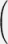P11 P12 P13 P21 P22 P23 P31 P32 P33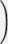• the origin shift (p) is:

•  p = ( p1 p2 p3 )

This transformation can be written in the following way:

(a', b', c') = (a, b, c)(P, p)

Example:
a' = b; b' = c + 1/4; c' = a + 1/4
The first column of the linear part of the transformation matrix is (0 1 0) and the first element of the origin shift is 0.
The second column of the linear part of the transformation matrix is (0 0 1) and the second element of the origin shift is 1/4 (or 0.25).
The third column of the linear part of the transformation matrix is (1 0 0) and the third element of the origin shift is 1/4 (or 0.25).

The matrix form of the transformation can be written in the following way:
• The linear part of the transformation matrix:

• P =0 0 1 1 0 0 0 1 0• The origin shift:

•  p = ( 0 1/4 1/4 )

### abc and xyz matrix notations

A (3x4) transformation matrix (P,p) with linear part P and the origin shift p can be represented by using the concise form:

P11a+P21b+P31c,P12a+P22b+P32c,P13a+P23b+P33c; p1,p2,p3

where it is understood that the abc entries should be read in columns.

As an example, a transformation matrix given as

-2/3a-1/3b+2/3c,-b,2a+b; 1/6,1/3,1/3

can be written as:

(P, p) =-2/3 0 2 1/6 -1/3 -1 1 1/3 2/3 0 0 1/3On the Bilbao Crystallographic Server, we also use the xyz notation likewise to represent transformation matrixes. But, this time, it should be interpreted in rows. Therefore, the example matrix above would be represented in the xyz notation as:

-2/3x+2z,-1/3x-y+z,2/3x; 1/6,1/3,1/3

This time we used the concise form:

P11x+P21y+P31z,P12x+P22y+P32z,P13x+P23y+P33z; p1,p2,p3

### Euler angles

According to Euler's rotation theorem, any rotation can be discribed using three angles (θ, φ, Ψ).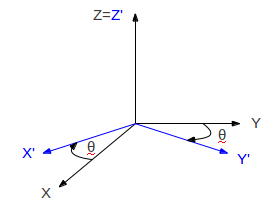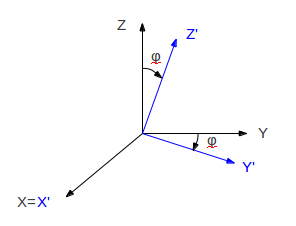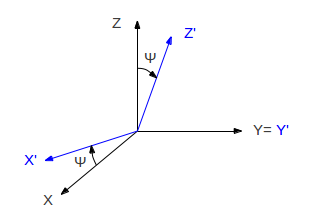A general rotation can be written as:

R =Cosθ -Sinθ 0 Sinθ Cosθ 0 0 0 11 0 0 0 Cosφ -Sinφ 0 Sinφ CosφCosΨ 0 SinΨ 0 1 0 -SinΨ 0 CosΨ[*] For more information: International Tables for Crystallography. Vol. A, Space Group Symmetry. Ed. Theo Hahn (3rd ed.), Dordrecht, Kluwer Academic Publishers, Section "Transformations in crystallography", 1995.